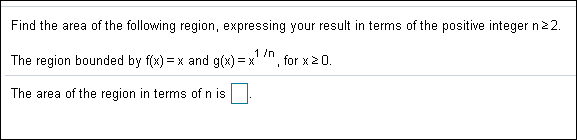# Find the area of the following region, expressing your result in terms of the positive integer n 2 2. 1 /n = x and g(x) =x The region bounded by f(x) for x 2 0. The area of the region in terms of n is

Questionhelp_outlineImage TranscriptioncloseFind the area of the following region, expressing your result in terms of the positive integer n 2 2. 1 /n = x and g(x) =x The region bounded by f(x) for x 2 0. The area of the region in terms of n is fullscreen

### Want to see this answer and more?

Experts are waiting 24/7 to provide step-by-step solutions in as fast as 30 minutes!*